# SSC (English Medium) 10th Standard Board Exam - Maharashtra State Board Important Questions for Geometry

Subjects
Topics
Subjects
Popular subjects
Topics
Geometry
< prev  1 to 20 of 567  next >

In the following figure, in ΔPQR, seg RS is the bisector of ∠PRQ. If PS = 6, SQ = 8, PR = 15, find QR.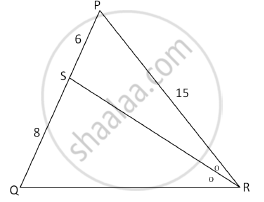Appears in 3 question papers
Chapter: [0.01] Similarity
Concept: Similarity of Triangles

In the following figure RP: PK= 3:2, then find the value of A(ΔTRP):A(ΔTPK).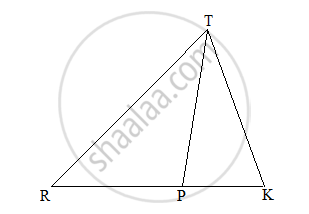Appears in 3 question papers
Chapter: [0.01] Similarity
Concept: Properties of Ratios of Areas of Two Triangles

Prove that ‘the opposite angles of a cyclic quadrilateral are supplementary’.

Appears in 3 question papers
Chapter: [0.03] Circle

If two circles with radii 8 cm and 3 cm, respectively, touch internally, then find the distance between their centers.

Appears in 3 question papers
Chapter: [0.03] Circle
Concept: Touching Circles

A person standing on the bank of river observes that the angle of elevation of the top of a tree standing on the opposite bank is 60°. When he moves 40 m away from the bank, he finds the angle of elevation to be 30°. Find the height of the tree and width of the river. (sqrt 3=1.73)

Appears in 3 question papers
Chapter: [0.06] Trigonometry
Concept: Heights and Distances

Using Euler’s formula, find V if E = 30, F = 12.

Appears in 3 question papers
Chapter: [0.07] Mensuration
Concept: Euler's Formula

Find the diagonal of a square whose side is 10 cm.

Appears in 3 question papers
Chapter: [0.07] Mensuration
Concept: Circumference of a Circle

In Fig. 3, AP and BP are tangents to a circle with centre O, such that AP = 5 cm and ∠APB = 60°. Find the length of chord AB.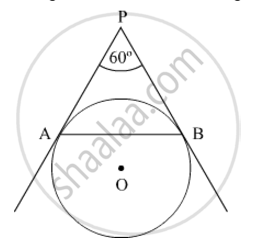Appears in 3 question papers
Chapter: [0.07] Mensuration
Concept: Areas of Sector and Segment of a Circle

A farmer connects a pipe of internal diameter 20 cm from a canal into a cylindrical tank which is 10 m in diameter and 2 m deep. If the water flows through the pipe at the rate of 4 km per hour, in how much time will the tank be filled completely?

Appears in 3 question papers
Chapter: [0.07] Mensuration
Concept: Surface Area of a Combination of Solids

In the following figure seg AB ⊥ seg BC, seg DC ⊥ seg BC. If AB = 2 and DC = 3, find (A(triangleABC))/(A(triangleDCB))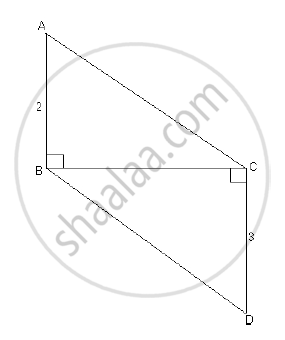Appears in 2 question papers
Chapter: [0.01] Similarity
Concept: Properties of Ratios of Areas of Two Triangles

Prove that the angle bisector of a triangle divides the side opposite to the angle in the ratio of the remaining sides.

Appears in 2 question papers
Chapter: [0.01] Similarity
Concept: Similarity of Triangles

In the following figure, seg BE ⊥ seg AB and seg BA ⊥ seg AD. If BE = 6 and $\text{AD} = 9 \text{ find} \frac{A\left( \Delta ABE \right)}{A\left( \Delta BAD \right)} \cdot$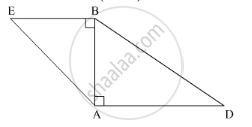Appears in 2 question papers
Chapter: [0.01] Similarity
Concept: Similarity of Triangles

In the given figure, AD is the bisector of the exterior ∠A of ∆ABC. Seg AD intersects the side BC produced in D. Prove that :

$\frac{BD}{CD} = \frac{AB}{AC}$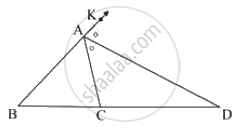Appears in 2 question papers
Chapter: [0.01] Similarity
Concept: Properties of Ratios of Areas of Two Triangles

ΔABP ~ ΔDEF and A(ΔABP) : A(ΔDEF) = 144:81, then AB : DE = ?

Appears in 2 question papers
Chapter: [0.01] Similarity
Concept: Similarity of Triangles

Find the height of an equilateral triangle whose side is 6 units.

Appears in 2 question papers
Chapter: [0.02] Pythagoras Theorem
Concept: Property of 30°- 60°- 90° Triangle Theorem

In ∆PQR, point S is the midpoint of side QR. If PQ = 11, PR = 17, PS = 13, find QR.

Appears in 2 question papers
Chapter: [0.02] Pythagoras Theorem
Concept: Right-angled Triangles and Pythagoras Property

Some question and their alternative answer are given.

In a right-angled triangle, if sum of the squares of the sides making right angle is 169 then what is the length of the hypotenuse?

Appears in 2 question papers
Chapter: [0.02] Pythagoras Theorem
Concept: Apollonius Theorem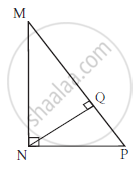In ΔMNP, ∠MNP = 90˚, seg NQ ⊥ seg MP, MQ = 9, QP = 4, find NQ.

Appears in 2 question papers
Chapter: [0.02] Pythagoras Theorem
Concept: Right-angled Triangles and Pythagoras Property

Prove that “The lengths of the two tangent segments to a circle drawn from an external point are equal.”

Appears in 2 question papers
Chapter: [0.03] Circle
Concept: Number of Tangents from a Point on a Circle

If two circles with radii 5 cm and 3 cm respectively touch internally, find the distance between their centres.

Appears in 2 question papers
Chapter: [0.03] Circle
Concept: Touching Circles
< prev  1 to 20 of 567  next >